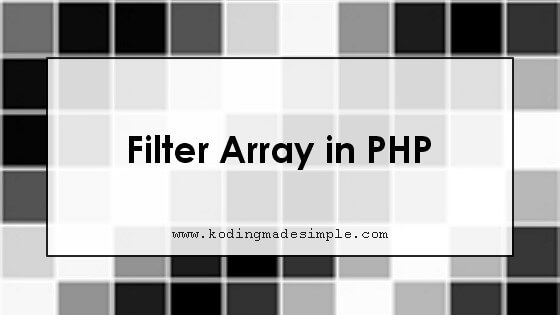# How to Filter Array by Value in PHP

Today let's see how to filter array by value in php. When working on a large data set, it would be useful to filter array values based on conditions. For this, you must iterate through the array, check each element against some condition and decide to keep it or discard it. It is a task that takes a lot of time to say.

Luckily you don't have to fuss over this. With PHP's native array_filter() function, you can filter the array using callback where you can specify the condition to filter each element of the array. The function returns the array containing elements that are successfully passed through the callback.## Using Array_Filter in PHP:

The function `array_filter()` filters elements of an array using a callback function.

```array_filter(array, callback, flag)
```

The function takes up three parameters of which the first one is mandatory and the rest others are optional.

1. array - The array to be filtered
2. callback - The callback function to use as a conditional filter
3. flag - Defines the arguments to be sent to the callback. To pass only key instead of value, set as ARRAY_FILTER_USE_KEY and use ARRAY_FILTER_USE_BOTH to pass both array key and value as arguments.

### Basic Array Filter Example:

We will see how the array filter works. Consider a basic array containing numbers from 1 to 10.

```array(1, 2, 3, 4, 5, 6, 7, 8, 9, 10)
```

Let's say we want to filter this array to contain only odd numbers. Below is the function to do it.

```<?php
\$result_array = array_filter(\$array, function (\$var) {
return(\$var & 1);
});
?>
```

Running our array through this function will produce an output like this,

```Array (  => 1  => 3  => 5  => 7  => 9 )
```

The function loops through each value of the source array, passes it to the callback, which in turn returns true if it is an odd number else false. If the callback returns true, that value is included in the result array.

If you want to use a separate function as a callback, you can do it this way,

```<?php
function filter_odd(\$var)
{
return(\$var & 1);
}

\$arr = array(1, 2, 3, 4, 5, 6, 7, 8, 9, 10);
print_r(array_filter(\$arr, "filter_odd"));

// output
// Array (  => 1  => 3  => 5  => 7  => 9 )
?>
```

### Filtering Associative Array:

While filtering, the function will also retain the array keys. Therefore, it's easier to filter associative arrays using it.

Consider this example,

```<?php
\$array = array(
'apple' => 'fruit',
'carrot' => 'vegetable',
'rose' => 'flower'
);

\$filtered_array = array_filter(\$array, function (\$var) {
return (\$var == 'flower');
});
print_r(\$filtered_array);

// produces output
// Array ( [rose] => flower )
?>
```

### Filtering Null Values in Array:

Filtering empty values in an array is even simpler with the help of the array filter. Remember that the callback parameter is optional? Now, consider this array,

```\$test = array(1, '', 'b', false, 'd', 2, null, true, 20)
```

Let's run this array through the filter function,

```<?php
print_r(array_filter(\$test));

// produces output
// Array (  => 1  => b  => d  => 2  => 1  => 20 )
?>
```

What happens here is, when you don't provide callback, the function will return those array elements which are true. So all the empty (null) values will be removed from the result array.

That explains about filtering array by values in php. In the same manner you can filter complex multidimensional array too. I hope this helps you. Please share the post if you find it useful :)